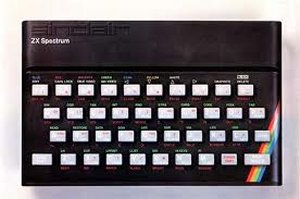Programming Variables

Variables? Probably time for a BASIC primer.

Let's just take apart a very simple program:

10 LET X=1
20 PRINT X
30 LET X=X+1
40 IF X<10 THEN GOTO 20
50 END

So, what does it all mean?

LET X=1 X is a variable or bucket. It is a place you can store or process numbers. X is a convenient name, it could have just as easily been Y, XY, MYVARIABLE or whatever. Every time you refer to X, the computer uses whatever number is stored there at the moment. In this case, LET X=1 means create a variable, call it X and set it initially to the value of 1. This is a NUMERIC variable which can store numbers. You could have a STRING variable to store characters, normally indicated by adding a \$ to the name, LET A\$="HELLO WORLD". You could also have BOOLEAN (True or False) or INTEGER (no decimal points) but all the same process.

That 10 bit meant this line comes before 11 and after 9 if either exist. 10 comes before 20 so the computer knows to run line 10 before it runs line 20. So why not make them 1,2,3,4 and 5? Because things were also added later. Imagine if I want a line LET Y=5? Easy:

15 LET Y=5

One computer, the Camputers Lynx, brought with it the option of line numbers as real numbers in which case you could have added:

1.5 LET Y=5.

That was quite unique though.

PRINT X Could not be simpler, Mr Computer, please put whatever is stored in my variable called X onto my screen. LPRINT X meant LINE PRINT - print to whatever printer I have connected.

What about that LET X=X+1 line? That is under BASIC Commands.Basic Commands.